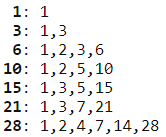# Problem 12 Highly divisible triangular number

The sequence of triangle numbers is generated by adding the natural numbers. So the 7th triangle number would be $1 + 2 + 3 + 4 + 5 + 6 + 7 = 28$. The first ten terms would be:

$$1, 3, 6, 10, 15, 21, 28, 36, 45, 55, …$$

Let us list the factors of the first seven triangle numbers:We can see that $28$ is the first triangle number to have over five divisors.
What is the value of the first triangle number to have over five hundred divisors?

# 问题 12 高度可除的三角数

$$1, 3, 6, 10, 15, 21, 28, 36, 45, 55, …$$# 思路分析

1 1 1
2 3 1+2=3
3 6 1+2+3=6
4 10 1+2+3+4=10
5 15 1+2+3+4+5=15
6 21 1+2+3+4+5+6=21
7 28 1+2+3+4+5+6+7=28

# 代码实现Thank you for your support to Jason ~微信支付宝